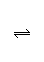# Thermochemistry: Play With Reaction Synthletor    Kelvin
 Haber ammonia synthesis+ 32Nitrogen+ HydrogenReactants AmmoniaProducts Products – Reactants ΔfHΔfHΣf-reactHΔfHΣf-prodHΣf-prodH- Σf-reactH= ΔrH(0.0 x 1) + (0.0 x 3) = 0.00 (-46.1 x 2) = -92.2 -92.2 - 0.0 = -92.2 kJmol-1 SSΣreactSSΣprodSΣprodS- ΣreactS= ΔrS(0.192 x 1) + (0.131 x 3) = 0.584 (0.192 x 2) = 0.385 0.385 - 0.584 = -0.199 kJK-1mol-1
 Gibbs free energy at 298K (standard conditions and standard temperature): ΔrG= ΔrH– (T298 x ΔrS)   =   -92.2 – (298 x -0.199)  = -33.0 kJmol-1
 ΔrH= -92.2 kJmol-1 Negative value: the reaction is exothermic ΔrS= -0.199 kJK-1mol-1 Negative value: the system becomes less dispersed ΔrG298K = -33.0 kJmol-1 Negative value: the reaction is feasible (spontaneous) Keq 298K = 6.07 x 105 Value is greater than 1: the reaction proceeds to the right ΔG° = 0.0, and the system is at equilibrium, at a temperature of 464K (191°C)

 Why Reactions Occur Timeline of Structural Theory

Queries, Suggestions, Bugs, Errors, Typos...

If you have any:

Queries